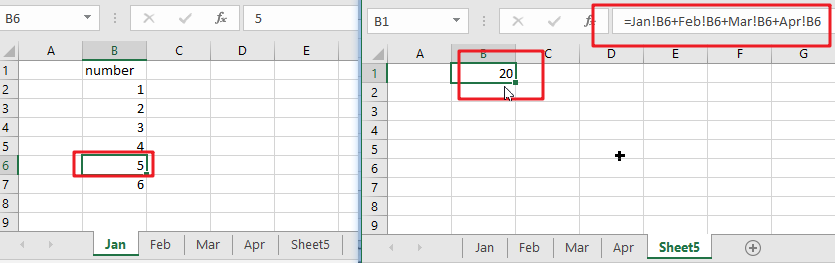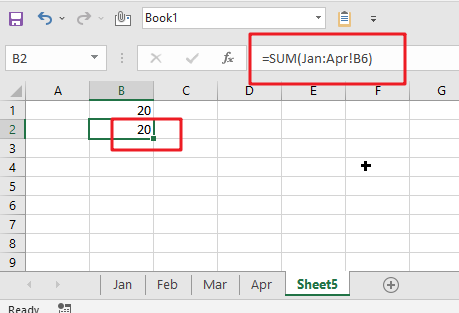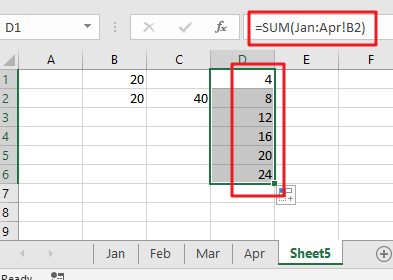# How to Use 3D SUM Multiple Worksheets

To sum a range of numbers is straightforward for most Excel users, but do you know how to establish a 3D reference to total the same range of numerous sheets. In this post, I will present the steps for this work. Let’s explore formulae to construct a 3D sum multiple worksheets in Excel.

## 3D SUM Multiple Worksheets in Microsoft Excel

The primary objective of this formula is to sum the same range in one or more sheets. Here we will learn how to construct a 3D SUM numerous spreadsheets in Microsoft Excel. That means, with the assistance of a formula based on the SUM function with the 3D reference you may able to sum the same range on one or more sheets. So, with the aid of this formula, you may able to build a 3D SUM numerous spreadsheets in Microsoft Excel.

## What is a 3D reference in Excel?

As indicated above, an Excel 3D reference enables you refer to the same cell or a range of cells in numerous spreadsheets. In other words, it addresses not just a range of cells, but also a range of worksheet names. The crucial thing is that all of the referred sheets should have the same pattern and the same data type. Please examine the following example.

Supposing you have monthly sales data on 4 separate sheets:

What you are seeking for is figuring out the grand total, i.e., adding up the sub-totals in four monthly sheets. The most apparent approach that comes to mind is sum up the sub-total cells from all the worksheets in the normal way:

`=Jan!B6+Feb! B6+Mar!B6+Apr!B6`However, what if you have 12 sheets for a whole year, or even many years’ worth of sheets? This would entail a significant amount of labor. Alternatively, you may use the SUM function in conjunction with a three-dimensional reference to sum across sheets:

`=SUM(Jan:Apr!B6)`This SUM formula does the same computations as the longer formula above, that is, it sums the values in cell B6 in all the sheets between the two border worksheets specified, in this case Jan and Apr.

Notes:

If you want to duplicate your three-dimensional formula to several cells and do not want the cell references to change, you may secure them by include the \$ symbol, i.e. by using absolute cell references such as:

` =SUM(Jan:Apr!\$B\$6)`

You are not even need to compute a sub-total in each monthly sheet; just mention the range of cells to be calculated in your 3D formula:

`=SUM(Jan:Apr!B2:B5)`

## Calculating a range of cells on many sheets using a 3D formula in Excel

If you wish to know the total sales for each particular product, create a summary table in which the items appear precisely as they do on monthly sheets, then enter the following three-dimensional formula in the top-most cell, B2 in this example:

=SUM(Jan:Apr!B2)Use a relative cell reference without the \$ symbol to ensure that the formula is modified for additional cells when copied along the column.

Let’s create a generic Excel 3D reference and formula based on the examples above.

### 3D SUM Multiple Worksheets General Formula:

`=SUM(Sheet1:Sheet3!A1)`

### Related Functions

• Excel SUM function
The Excel SUM function will adds all numbers in a range of cells and returns the sum of these values. You can add individual values, cell references or ranges in excel.The syntax of the SUM function is as below:= SUM(number1,[number2],…)…
Related Posts

Calculate Cumulative Totals with Excel SUM Function

Today, through a simple example, we will show you how to use one of the most common-used Mathematical functions in excel, the SUM function, to add up the sum. In our daily life, we keep an account of what we ...

Check Dates in chronological order

Assume you have a date list that has different date formats, as seen in the accompanying picture. In this instance, Excel's Sort function will fail to sort them appropriately. However, you may convert all various date formats to a particular ...

If Cell Begins with One of Three Supplied Characters

If you want to test values to see if they begin with some given specific characters like “x”, ”y”, or “z”, you can create a formula with COUNTIF and SUM functions to return results. EXAMPLE You can see “TRUE” or ...

Average per Week by Formula in Excel

We usually apply AVERAGE function or relevant functions to return average directly in Excel worksheet. But in some situations, only applying average relevant functions cannot figure out our problem. Sometimes we can create a formula with functions and mathematical operation ...

How to Sum if Contains an Asterisk

To add numbers together we need to apply SUM function. And if we want to add numbers based on some conditions, we can add criteria with the help of SUMIFS function, SUMIFS can filter data with multiple criteria effectively. If ...

How to Sum in Vertical Range

If we want to add numbers based on some conditions in Excel worksheet, we can add criteria with the help of SUMIFS function, SUMIFS can filter data with multiple criteria effectively. In this article, we will introduce you the method ...

How to Sum in Horizontal Range

To add numbers together we need to apply SUM function. But if we want to add numbers based on some conditions, we can add criteria with the help of SUMIFS function, SUMIFS can filter data with multiple criteria effectively. In ...

How to Sum with Criteria and Or Logic in Excel

To add numbers together we need to apply SUM function. And if we want to add numbers based on some conditions, we can add criteria with the help of SUMIFS function, SUMIFS can filter data with multiple criteria effectively. If ...

How To Sum the Largest N Values in Excel

Sometimes we may want to sum the largest N numbers or top N numbers in a range. In this article, we will show you the method of “SUM the Largest N Numbers” by a simple formula which consist of SUMPRODUCT ...

How to Sum for Cell Contains Formula Only in Excel

Sometimes values are created by formulas in cells. If we want to sum values which are created by formulas from a range, but some values which are hardcoded also list in the same range, how can we filter out matched ...

Sidebar